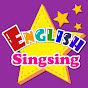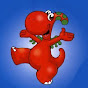# Practice English Speaking&Listening with: Java #02 - Basic Types and Objects

Normal
(0)
Difficulty: 0

Java has eight basic (or primitive) types.

The Java class BasicTypes shows these. They are:

Int an integer (that is, positive or negative whole number).

The int type has 32 bits,

so it can take on values from negative two to the 31 to

positive two to the 31 minus one.

Float a real number.

This is called a float because the decimal point is said to

float in the number.

(This means that there isnt fixed precision after the decimal point.

Small numbers have more digits of precision after the

decimal point than big ones).

Double was so named because it has 64 bits,

or double the precision of float.

Char represents a single character.

Here we use single quotes to assign the character C to the variable.

A String object is a sequence of characters,

and a string constant uses double quotes.

The Boolean type is used to represent truth values

(either true or false).

Just as double and float are different sizes of real numbers,

Java also has different sizes of integers.

Byte, short and long are 8, 16 and 64 bit integers, respectively.

The next line gives the variable gallons the value of 7.

Since we told Java above that gallons is an int,

we dont need to say that again.

Each variable should be declared only once,

and it is useful to group all of these at the top of the method.

Note that we refer to gallons as a variable,

meaning its value can change.

It is important not to think of the equal sign in Java as a math equation.

It is instead called an assignment.

The next assignment highlights the difference.

As an equation, gallons equals gallons plus one would have no solution.

As an assignment, it says, evaluate the right side

and make that the new value of the variable gallons.

So, we add one to gallons and it becomes eight.

At the end, we print out gallons per mile.

Note that we can use to append the value of gallons_per_mile

to the end of the string.

If we compile and run this program, it says that gallons (8),

divided by miles (450) is zero!

This is because when Java divides two integers, it gives an integer result.

(It gets rid of everything after the decimal point,

even though the final answer will be put in a double.)

To fix this, we can add a typecast, making the

value of gallons into a double before doing the division.

After we do this, we see the correct result of the division.

Variables of basic types behave differently than

variables whose type is a class.

In the class BasicTypesVersusObjects, we declare

two different double variables, double1 and double2.

We set double2 to equal double 1, then add 5 to double1.

When we print out the values of double1 and double2,

you will notice that the value of double1 changed,

but the value of double2 is still 31.

That is because assignment for basic types does a copy.

Objects, variables of a class, behave differently.

We also declare two points, p and p2.

Although we can assign constant values directly to the doubles,

we must call a constructor (indicated by the keyword new)

to create an object of the class.

If we fail to do so, well either get a compiler error,

or a NullPointerException at runtime.

When we assign P2 to the value P, we do NOT make a copy.

Instead, P and P2 now both reference the same object.

As a result, when we move the point p by calling

the translate method on it, P2 also moves.

The Description of Java #02 - Basic Types and Objects

### Channels for learning English

•English Singsing
•Gogo Lessons & English with Games
•Boston English Centre
•Learn English With TV Series
•Listen English Everyday
•Daily English Conversation
•English Speeches
•Langfocus